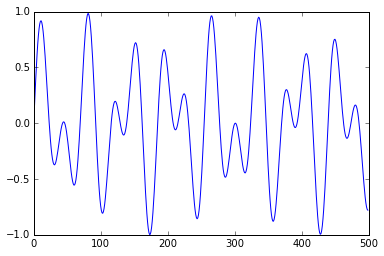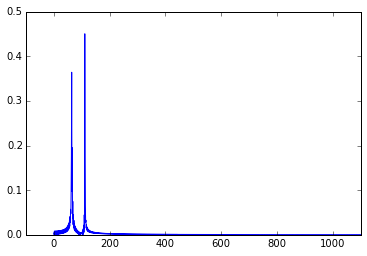# Audio Synthesis with Sines

In :
import numpy as np
import numpy.linalg as la
import matplotlib.pyplot as pt

In :
from html5_audio import DEFAULT_RATE, get_html5_wave_player


Let's fix some parameters. The sample_rate says how many values (so-called "samples") are played per second.

In :
sample_rate = DEFAULT_RATE
sample_rate

Out:
44100

In :
t = np.linspace(0, 1, sample_rate)


Here are some example tones. What are they?

In :
#signal = np.sin(440*2*np.pi*t)
#signal = np.sin(880*2*np.pi*t)
#signal = 0.5 * (np.sin(880*2*np.pi*t) + np.sin(440*2*np.pi*t))
signal = 0.5 * (np.sin(1209*2*np.pi*t) + np.sin(697*2*np.pi*t))

In :
get_html5_wave_player(signal)

Out:
In :
pt.plot(signal[:500])

Out:
[<matplotlib.lines.Line2D at 0x7f9bcc371e10>]That last tone--wouldn't it be useful to pick that apart into its frequency components?

In :
n = 2047
assert n % 2 == 1

cos_k = np.arange(0, n//2 + 1, dtype=np.float64)
sin_k = np.arange(1, n//2 + 1, dtype=np.float64)

x = np.linspace(0, 2*np.pi, n, endpoint=False)

DFT = np.zeros((n,n))
DFT[:, ::2] = np.cos(cos_k*x[:, np.newaxis])
DFT[:, 1::2] = np.sin(sin_k*x[:, np.newaxis])

IDFT = la.inv(DFT)

In :
pt.xlim([-100, 1100])
pt.plot(np.abs(IDFT.dot(signal[:n])))

Out:
[<matplotlib.lines.Line2D at 0x7f9bcc2d6860>]In []: